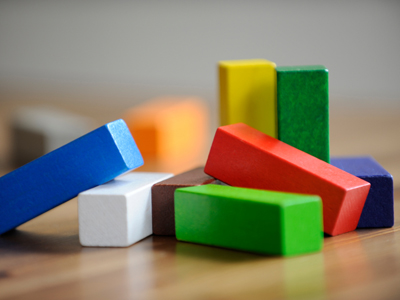Six wooden bricks weigh 600g. 2 bricks weigh 200g.

# Solving Problems (Year 3)

Solving problems is the very essence of Maths at every level, including KS2. In Year Three children will be shown how to arrive at the correct solution to simple problems based on real life scenarios by using addition, subtraction, multiplication and division.

Solving problems gets you using your brain and tests your thinking skills. Solving real life problems can involve numbers and measures of time, length, capacity and weight. You can use all the operations of addition, subtraction, multiplication and division to help you find the right answers - you just need to work out which!

Put your thinking cap on and have a go at playing this quiz to find out how well you can solve real life problems by using addition, subtraction, multiplication and division.

1.
I think of a number, double it and add 3. The answer is 45. What was my first number?
21
22
23
25
You can work this one out by doing the problem backwards:
45 - 3 = 42
42 ÷ 2 = 21
2.
There are three glasses of water containing 350ml, 250ml and 400ml. When pouring the water into a bowl, half of it is spilt. How much water is left?
400ml
450ml
500ml
550ml
To solve this problem you need to add 350ml + 250ml + 400ml = 1,000ml. Half of 1,000ml is 500ml
3.
Six wooden bricks weigh 600g. What do 2 bricks weigh?
100g
200g
250g
300g
To calculate you need to find how much one brick weighs so 600g ÷ 6 = 100g, so 2 bricks weigh 200g
4.
Richard went jogging at 3:30. He jogged for 30 minutes, walked for 10 minutes then jogged again. He arrived home at 4:20. How long was his second jog?
10 minutes
15 minutes
20 minutes
25 minutes
If you add 30 minutes to 3:30 it will be 4:00; add another 10 minutes and it becomes 4:10, so 4:20 is 10 more minutes
5.
An apple weighs 25g. How much would 4 apples weigh?
50g
75g
100g
125g
25 x 4 = 100 so 4 apples would weigh 100g
6.
I think of a number and subtract 12. The answer is 15. What was my first number?
3
5
27
29
You can work out the answer by adding 15 and 12 together
7.
Two pieces of string are 10cm long and three pieces of string are 6cm long. What is the total length?
18cm
20cm
38cm
40cm
10 x 2 = 20 and 6 x 3 = 18
20 + 18 = 38
8.
There are 25ml of medicine in a bottle. A teaspoon holds 5ml. How many teaspoons of medicine are in the bottle?
2
3
4
5
The way to work this one out is to divide 25 by 5
9.
A piece of string is 16cm. I cut off 7cm. How long is my string now?
7cm
9cm
10cm
12cm
16 - 7 = 9 so the string is 9cm long
10.
A cake goes in the oven at 12 o’clock. It bakes for 35 minutes. What time did the cake come out of the oven?
Twenty-five past twelve
Twenty-five to twelve
Twenty-five to one
Twenty-five past one
35 minutes after 12 o'clock is 12:35 or twenty-five to one